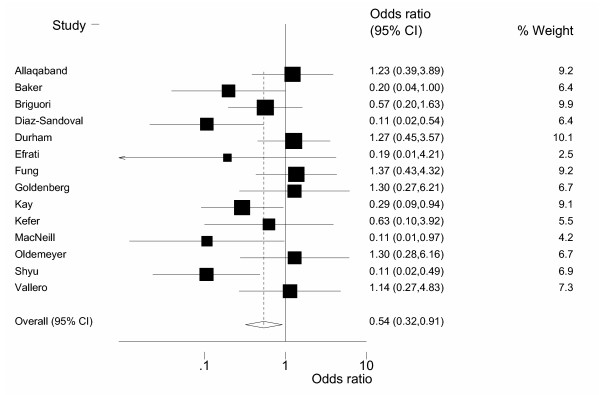# Forest plots

## 2005-01-12

Many meta-analyses use a graph known as a forest plot. I was always confused by the funny squares in a forest plot, so I looked for a description. An example of a forest plot appears in

and because this is an open-access article, I can reproduce the graph here.Here is what the User’s Guide for RevMan (software created by the Cochrane Collaboration) says about forest plots:

The graph is a forest plot where the confidence interval (CI) for each study is represented by a horizontal line and the point estimate is represented by a square. The size of the square corresponds to the weight of the study in the meta-analysis. The confidence interval for totals are represented by a diamond shape. The scale used on the graph depends on the statistical method. Dichotomous data (except for risk differences) are displayed on a logarithmic scale. Continuous data and risk differences are displayed on a linear scale. Generic inverse variance data are displayed on either a logarithmic scale or a linear scale depending on the settings in RevMan.http://www.cc-ims.net/download/revman/Documentation/User%20guide.pdf (page 36).

You can find an earlier version of this page on my original website.